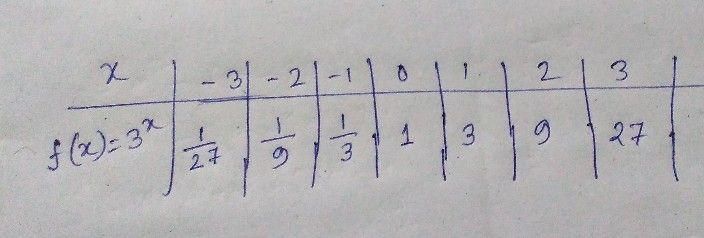Symbol
ProblemCan You Show Me the Way? Complete the table of values of the exponential function. Then, draw its graph in the given coordinate plane. $x$ $-3$ $-2$ $-1$ $0$ $1$ $2$ $3$ $f\left(x\right)=3x$
10th-13th grade
Other
Search count: 109
SolutionQanda teacher - Liza